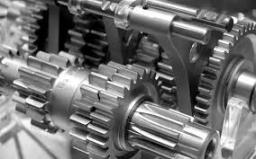# Machines 2

Two machines for 50 hours produce 2,000 products. How many machines need to buy to make in 30 hours 15,000 products?

n =  23

### Step-by-step explanation:

30·(2000/2/50)·(n+2)=15000

30000n = 690000

n = 690000/30000 = 23

n = 23

Our simple equation calculator calculates it.Did you find an error or inaccuracy? Feel free to write us. Thank you!

Tips for related online calculators
Do you have a linear equation or system of equations and looking for its solution? Or do you have a quadratic equation?
Do you want to convert time units like minutes to seconds?